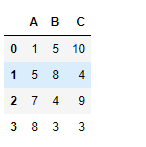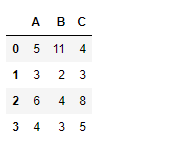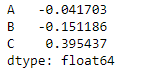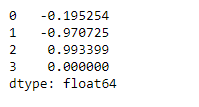# Python | Pandas dataframe.corrwith()

Python is a great language for doing data analysis, primarily because of the fantastic ecosystem of data-centric python packages. Pandasis one of those packages and makes importing and analyzing data much easier.

Pandas` dataframe.corrwith()` is used to compute pairwise correlation between rows or columns of two DataFrame objects. If the shape of two dataframe object is not same then the corresponding correlation value will be a `NaN` value.

Syntax: DataFrame.count(axis=0, level=None, numeric_only=False)

Parameters:
other : DataFrame
axis : 0 or ‘index’ to compute column-wise, 1 or ‘columns’ for row-wise
drop : Drop missing indices from result, default returns union of all

Returns: correls : Series

Note: The correlation of a variable with itself is 1.

Example #1: Use `corrwith()` function to find the correlation among two dataframe objects along the column axis

 `# importing pandas as pd ` `import` `pandas as pd ` ` `  `# Creating the first dataframe ` `df1 ``=` `pd.DataFrame({``"A"``:[``1``, ``5``, ``7``, ``8``],  ` `                    ``"B"``:[``5``, ``8``, ``4``, ``3``], ` `                    ``"C"``:[``10``, ``4``, ``9``, ``3``]}) ` ` `  `# Creating the second dataframe  ` `df2 ``=` `pd.DataFrame({``"A"``:[``5``, ``3``, ``6``, ``4``], ` `                    ``"B"``:[``11``, ``2``, ``4``, ``3``], ` `                    ``"C"``:[``4``, ``3``, ``8``, ``5``]}) ` ` `  `# Print the first dataframe ` `print``(df1, ``"\n"``) ` ` `  `# Print the second dataframe ` `print``(df2) `Now find the correlation among the columns of the two data frames along the row axis.

 `# To find the correlation among the ` `# columns of df1 and df2 along the column axis ` `df1.corrwith(df2, axis ``=` `0``) `

Output :The output series contains the correlation between the three columns of two dataframe objects respectively.

Example #2: Use `corrwith()` function to find the correlation among two dataframe objects along the row axis

 `# importing pandas as pd ` `import` `pandas as pd ` ` `  `# Creating the first dataframe ` `df1 ``=` `pd.DataFrame({``"A"``:[``1``, ``5``, ``7``, ``8``], ` `                    ``"B"``:[``5``, ``8``, ``4``, ``3``], ` `                    ``"C"``:[``10``, ``4``, ``9``, ``3``]}) ` ` `  `# Creating the second dataframe  ` `df2 ``=` `pd.DataFrame({``"A"``:[``5``, ``3``, ``6``, ``4``], ` `                    ``"B"``:[``11``, ``2``, ``4``, ``3``],  ` `                    ``"C"``:[``4``, ``3``, ``8``, ``5``]}) ` ` `  `# To find the correlation among the ` `# columns of df1 and df2 along the row axis ` `df1.corrwith(df2, axis ``=` `1``) `

Output :The output series contains the correlation between the four rows of two data frame objects respectively.

Whether you're preparing for your first job interview or aiming to upskill in this ever-evolving tech landscape, GeeksforGeeks Courses are your key to success. We provide top-quality content at affordable prices, all geared towards accelerating your growth in a time-bound manner. Join the millions we've already empowered, and we're here to do the same for you. Don't miss out - check it out now!